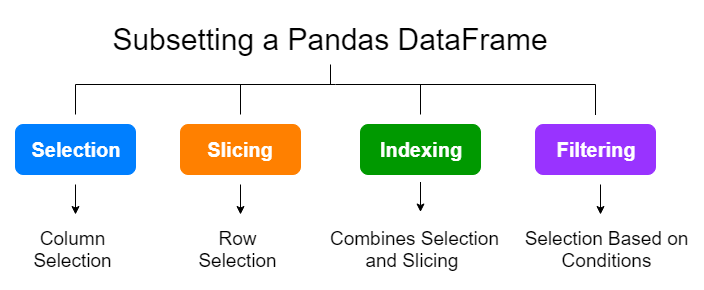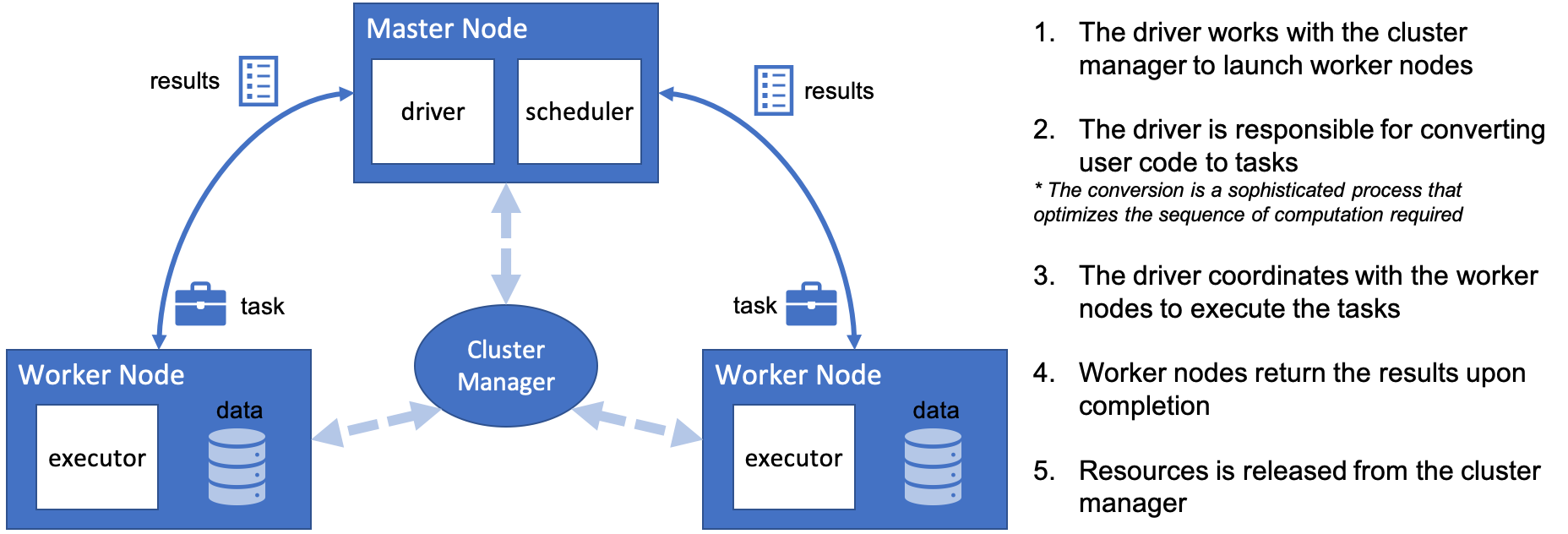1623175620

# 23 Efficient Ways of Subsetting a Pandas DataFrame

### With Selection, Slicing, Indexing and Filtering

In part 1 and part 2, we’ve learned how to inspect, describe and summarize a Pandas DataFrame. Today, we’ll learn how to extract a subset of a Pandas DataFrame. This is very useful because we often want to perform operations on subsets of our data. There are many different ways of subsetting a Pandas DataFrame. You may need to select specific columns with all rows. Sometimes, you want to select specific rows with all columns or select rows and columns that meet a specific criterion, etc.

All different ways of subsetting can be divided into 4 categories: SelectionSlicing, **Indexing **and Filtering.(Image by author)

As you continue reading this post, you’ll learn the differences between these categories.

Before discussing any of the methods of subsetting a data frame, it is worth distinguishing between a Pandas Series object and a Pandas DataFrame object.

#programming #python #pandas #efficient ways of subsetting a pandas dataframe #pandas dataframe #subset

## Buddha Community1623175620

## 23 Efficient Ways of Subsetting a Pandas DataFrame

### With Selection, Slicing, Indexing and Filtering

In part 1 and part 2, we’ve learned how to inspect, describe and summarize a Pandas DataFrame. Today, we’ll learn how to extract a subset of a Pandas DataFrame. This is very useful because we often want to perform operations on subsets of our data. There are many different ways of subsetting a Pandas DataFrame. You may need to select specific columns with all rows. Sometimes, you want to select specific rows with all columns or select rows and columns that meet a specific criterion, etc.

All different ways of subsetting can be divided into 4 categories: SelectionSlicing, **Indexing **and Filtering.(Image by author)

As you continue reading this post, you’ll learn the differences between these categories.

Before discussing any of the methods of subsetting a data frame, it is worth distinguishing between a Pandas Series object and a Pandas DataFrame object.

#programming #python #pandas #efficient ways of subsetting a pandas dataframe #pandas dataframe #subset1623927960

## Pandas DataFrame vs. Spark DataFrame: When Parallel Computing Matters

### With Performance Comparison Analysis and Guided Example of Animated 3D Wireframe Plot

Python is famous for its vast selection of libraries and resources from the open-source community. As a Data Analyst/Engineer/Scientist, one might be familiar with popular packages such as NumpyPandasScikit-learnKeras, and TensorFlow. Together these modules help us extract value out of data and propels the field of analytics. As data continue to become larger and more complex, one other element to consider is a framework dedicated to processing Big Data, such as Apache Spark. In this article, I will demonstrate the capabilities of distributed/cluster computing and present a comparison between the Pandas DataFrame and Spark DataFrame. My hope is to provide more conviction on choosing the right implementation.

### Pandas DataFrame

Pandas has become very popular for its ease of use. It utilizes DataFrames to present data in tabular format like a spreadsheet with rows and columns. Importantly, it has very intuitive methods to perform common analytical tasks and a relatively flat learning curve. It loads all of the data into memory on a single machine (one node) for rapid execution. While the Pandas DataFrame has proven to be tremendously powerful in manipulating data, it does have its limits. With data growing at an exponentially rate, complex data processing becomes expensive to handle and causes performance degradation. These operations require parallelization and distributed computing, which the Pandas DataFrame does not support.

### Introducing Cluster/Distribution Computing and Spark DataFrame

Apache Spark is an open-source cluster computing framework. With cluster computing, data processing is distributed and performed in parallel by multiple nodes. This is recognized as the MapReduce framework because the division of labor can usually be characterized by sets of the mapshuffle, and reduce operations found in functional programming. Spark’s implementation of cluster computing is unique because processes 1) are executed in-memory and 2) build up a query plan which does not execute until necessary (known as lazy execution). Although Spark’s cluster computing framework has a broad range of utility, we only look at the Spark DataFrame for the purpose of this article. Similar to those found in Pandas, the Spark DataFrame has intuitive APIs, making it easy to implement.#pandas dataframe vs. spark dataframe: when parallel computing matters #pandas #pandas dataframe #pandas dataframe vs. spark dataframe #spark #when parallel computing matters1623183300

## 9 Efficient Ways for Describing and Summarizing a Pandas DataFrame

### A guide to using pandas effectively and efficiently — Part 2

In part 1 of “A guide to using pandas effectively and efficiently”  article series, we’ve discussed 10 efficient ways of examing the structure  of a Pandas DataFrame object. If you haven’t read that post, please read it before continuing to read this one. Here is the link:

Still, we don’t know anything about the data in the DataFrame. In this post, we’ll discuss numerical and graphical methods commonly used to describe and summarise a Pandas DataFrame.

First, we’ll begin with numerical methods

## Graphical methods for describing and summarizing a Pandas DataFrame

#data-science #pandas #tehnology #python #pandas dataframe #efficient ways for describing and summarizing a pandas dataframe1623922440

## 8 Ways to Filter Pandas Dataframes

### A practical guide for efficient data analysis

Pandas is a popular data analysis and manipulation library for Python. The core data structure of Pandas is dataframe which stores data in tabular form with labelled rows and columns.

A common operation in data analysis is to filter values based on a condition or multiple conditions. Pandas provides a variety of ways to filter data points (i.e. rows). In this article, we will cover 8 different ways to filter a dataframe.

We start by importing the libraries.

``````import numpy as np
import pandas as pd
``````

Let’s create a sample dataframe for the examples.

``````df = pd.DataFrame({

name':['Jane','John','Ashley','Mike','Emily','Jack','Catlin'],
'ctg':['A','A','C','B','B','C','B'],
'val':np.random.random(7).round(2),
'val2':np.random.randint(1,10, size=7)
})
``````

#python #programming #data-science #ways to filter pandas dataframes #filter pandas dataframes #pandas dataframes1623370500

## Practice Problems: How To Join DataFrames in Pandas

Hey - Nick here! This page is a free excerpt from my \$199 course Python for Finance, which is 50% off for the next 50 students.

It’s now time for some practice problems! See below for details on how to proceed.

### Course Repository & Practice Problems

All of the code for this course’s practice problems can be found in this GitHub repository.

There are two options that you can use to complete the practice problems:

• Open them in your browser with a platform called Binder using this link (recommended)
• Download the repository to your local computer and open them in a Jupyter Notebook using Anaconda (a bit more tedious)

Note that binder can take up to a minute to load the repository, so please be patient.

Within that repository, there is a folder called `starter-files` and a folder called `finished-files`. You should open the appropriate practice problems within the `starter-files` folder and only consult the corresponding file in the `finished-files` folder if you get stuck.

The repository is public, which means that you can suggest changes using a pull request later in this course if you’d like.

#dataframes #pandas #practice problems: how to join dataframes in pandas #how to join dataframes in pandas #practice #/pandas/issues.